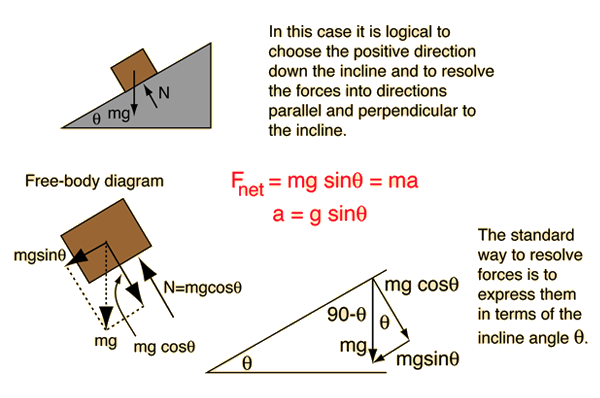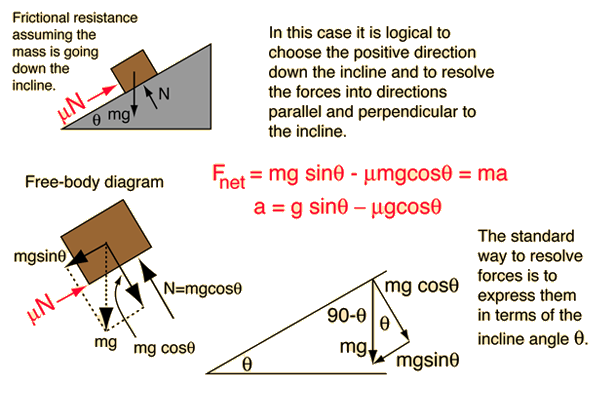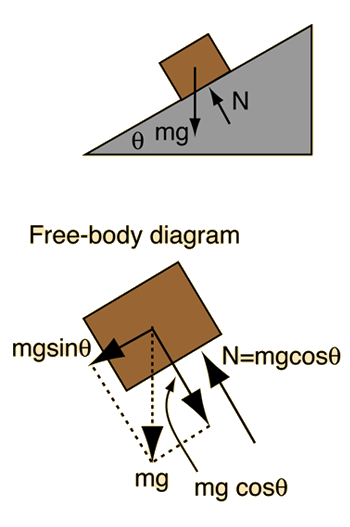# Mass on Frictionless InclineOne of the insights that comes from the setup of this problem is that the force required to push a mass m up a frictionless incline is equal to mgsinθ. Checking the limiting cases, you find that it takes no force along a horizontal frictionless surface (θ=0), and a force = mg on a vertical frictionless surface (θ=90°) as you would expect.

### Calculation

Index

Newton's laws

Standard mechanics problems

 HyperPhysics***** Mechanics R Nave
Go Back

# Mass on Incline With Friction### Remove friction

Index

Newton's laws

Standard mechanics problems

 HyperPhysics***** Mechanics R Nave
Go Back

# Mass on Frictionless InclineApplication of Newton's second law to mass on incline.
For a frictionless incline of angle degrees, the acceleration is given by the acceleration of gravity times the sine of the angle.

### Acceleration =m/s2

compared to 9.8 m/s² for freefall. If the height of the incline is h=m, then the time to slide down the incline from rest would be t=seconds, compared to a time of t=seconds to drop from that height. The speed at the bottom of the incline would be m/s. These calculations can be done with the motion equations.
Note that the speed at the bottom of a frictionless incline does not depend upon the angle of the incline! It just takes longer to get up to that speed for a smaller angle of incline.